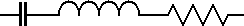Capacitor Inductor and Resistor in Series Calculator

This calculator finds the complex impedance (real and imaginary imaginary values) of a capacitor and an inductor in series.Frequency: MHz kHz Hz Capacitance: uF nF pF Inductance: mH uH nH Resistance: MΩ kΩ Ω Z =

The complex impedance (Z) (real and imaginary, or inductance and reactance) of a capacitor, inductor and resistor in series at a particular frequency can be calculated using the following formula.Where:

f is the Frequency in Hz

C is the Capacitance in Farads

L is the Inductance in Henries

R is the Resistance in Ohms

XC is the Capacitive Reactance in Ohms

XL is the Inductive Reactance in Ohms

This calculator uses JavaScript and will function in most modern browsers. For more information see About our calculators

This calculator is provided free by Chemandy Electronics in order to promote the FLEXI-BOX